What does it do?

Converts a number from one measurement system to another

Formula breakdown:

=CONVERT(number, from_unit, to_unit)

What it means:

=CONVERT(number, starting measurement system, target measurement system)

Did you know that Excel can convert numbers from one measurement system to another measurement system? Yes you can with the CONVERT Formula! You will be surprised at what units it can convert for you!

I explain how you can do this below:STEP 1: We need to enter the CONVERT function in a blank cell:

## =CONVERT(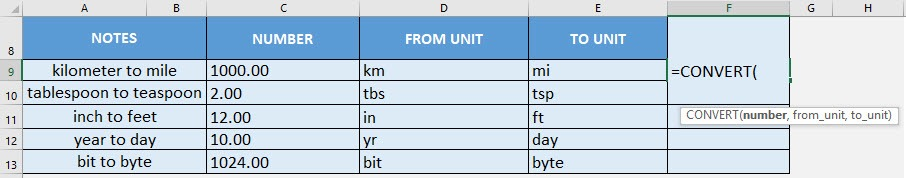STEP 2: The CONVERT arguments:

## number

What is the numeric measurement?

Select the cell containing the number that you want to convert

## =CONVERT(C9,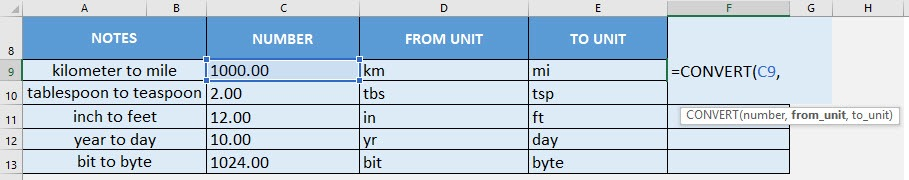## from_unit

What is the starting measurement system?

Select the cell containing the starting measurement system.

## =CONVERT(C9, D9,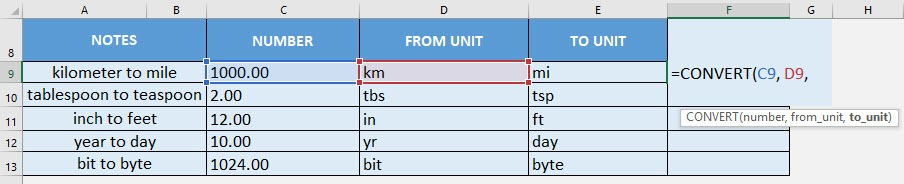## to_unit

What is the target measurement system?

Select the cell containing the target measurement system.

## =CONVERT(C9, D9, E9)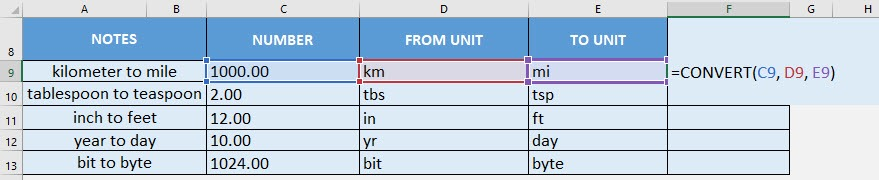Apply the same formula to the rest of the cells by dragging the lower right corner downwards.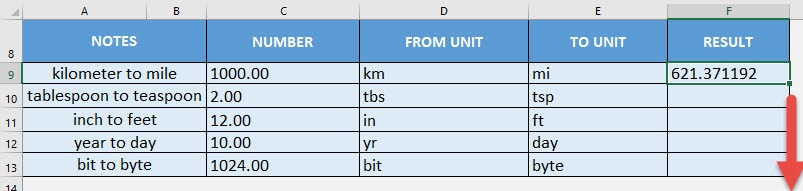You now have your conversions completed!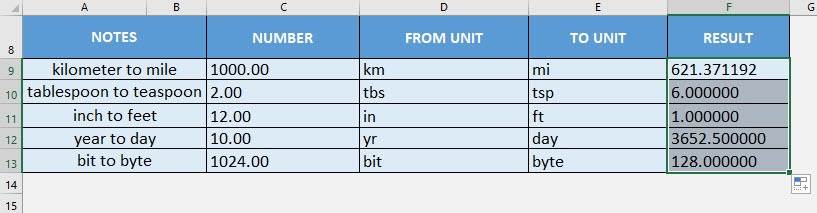Here is an extensive list of all of the units that you can use:

 Category Unit Usage in CONVERT FORMULA Weight and mass Gram “g” Weight and mass Slug “sg” Weight and mass Pound mass (avoirdupois) “lbm” Weight and mass U (atomic mass unit) “u” Weight and mass Ounce mass (avoirdupois) “ozm” Weight and mass Grain “grain” Weight and mass U.S. (short) hundredweight “cwt” or “shweight” Weight and mass Imperial hundredweight “uk_cwt” or “lcwt” (“hweight”) Weight and mass Stone “stone” Weight and mass Ton “ton” Weight and mass Imperial ton “uk_ton” or “LTON” (“brton”) Distance Meter “m” Distance Statute mile “mi” Distance Nautical mile “Nmi” Distance Inch “in” Distance Foot “ft” Distance Yard “yd” Distance Angstrom “ang” Distance Ell “ell” Distance Light-year “ly” Distance Parsec “parsec” or “pc” Distance Pica (1/72 inch) “Picapt” or “Pica” Distance Pica (1/6 inch) “pica” Distance U.S survey mile (statute mile) “survey_mi” Time Year “yr” Time Day “day” or “d” Time Hour “hr” Time Minute “mn” or “min” Time Second “sec” or “s” Pressure Pascal “Pa” (or “p”) Pressure Atmosphere “atm” (or “at”) Pressure mm of Mercury “mmHg” Pressure PSI “psi” Pressure Torr “Torr” Force Newton “N” Force Dyne “dyn” (or “dy”) Force Pound force “lbf” Force Pond “pond” Energy Joule “J” Energy Erg “e” Energy Thermodynamic calorie “c” Energy IT calorie “cal” Energy Electron volt “eV” (or “ev”) Energy Horsepower-hour “HPh” (or “hh”) Energy Watt-hour “Wh” (or “wh”) Energy Foot-pound “flb” Energy BTU “BTU” (or “btu”) Power Horsepower “HP” (or “h”) Power Pferdestärke “PS” Power Watt “W” (or “w”) Magnetism Tesla “T” Magnetism Gauss “ga” Temperature Degree Celsius “C” (or “cel”) Temperature Degree Fahrenheit “F” (or “fah”) Temperature Kelvin “K” (or “kel”) Temperature Degrees Rankine “Rank” Temperature Degrees Réaumur “Reau” Volume Teaspoon “tsp” Volume Modern teaspoon “tspm” Volume Tablespoon “tbs” Volume Fluid ounce “oz” Volume Cup “cup” Volume U.S. pint “pt” (or “us_pt”) Volume U.K. pint “uk_pt” Volume Quart “qt” Volume Imperial quart (U.K.) “uk_qt” Volume Gallon “gal” Volume Imperial gallon (U.K.) “uk_gal” Volume Liter “l” or “L” (“lt”) Volume Cubic angstrom “ang3” or “ang^3” Volume U.S. oil barrel “barrel” Volume U.S. bushel “bushel” Volume Cubic feet “ft3” or “ft^3” Volume Cubic inch “in3” or “in^3” Volume Cubic light-year “ly3” or “ly^3” Volume Cubic meter “m3” or “m^3” Volume Cubic Mile “mi3” or “mi^3” Volume Cubic yard “yd3” or “yd^3” Volume Cubic nautical mile “Nmi3” or “Nmi^3” Volume Cubic Pica “Picapt3”, “Picapt^3”, “Pica3” or “Pica^3” Volume Gross Registered Ton “GRT” (“regton”) Volume Measurement ton (freight ton) “MTON” Area International acre “uk_acre” Area U.S. survey/statute acre “us_acre” Area Square angstrom “ang2″ or “ang^2” Area Are “ar” Area Square feet “ft2” or “ft^2” Area Hectare “ha” Area Square inches “in2” or “in^2” Area Square light-year “ly2” or “ly^2” Area Square meters “m2” or “m^2” Area Morgen “Morgen” Area Square miles “mi2” or “mi^2” Area Square nautical miles “Nmi2” or “Nmi^2” Area Square Pica “Picapt2”, “Pica2”, “Pica^2” or “Picapt^2” Area Square yards “yd2” or “yd^2” Information Bit “bit” Information Byte “byte” Speed Admiralty knot “admkn” Speed Knot “kn” Speed Meters per hour “m/h” or “m/hr” Speed Meters per second “m/s” or “m/sec” Speed Miles per hour “mph”

The following abbreviated unit prefixes can be added in front of any metric from_unit or to_unit.

 Prefix Multiplier Abbreviation yotta 1.00E+24 “Y” zetta 1.00E+21 “Z” exa 1.00E+18 “E” peta 1.00E+15 “P” tera 1.00E+12 “T” giga 1.00E+09 “G” mega 1.00E+06 “M” kilo 1.00E+03 “k” hecto 1.00E+02 “h” dekao 1.00E+01 “da” or “e” deci 1.00E-01 “d” centi 1.00E-02 “c” milli 1.00E-03 “m” micro 1.00E-06 “u” nano 1.00E-09 “n” pico 1.00E-12 “p” femto 1.00E-15 “f” atto 1.00E-18 “a” zepto 1.00E-21 “z” yocto 1.00E-24 “y”

How to Use the CONVERT Formula in Excel